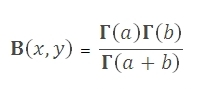# SQL Server BETA function

Updated: 9 August 2010

Use BETA to calculate the beta function. This is equivalent of Beta[a, b] in Mathematica. Also called the Euler integral, the BETA function is defined byThe beta function is symmetric, meaningSyntax
SELECT [wctStatistics].[wct].[BETA] (
<@A, float,>
,<@B, float,>)
Arguments
@A
is any real number. @A is an expression of type float or of a type that can be implicitly converted to float
@B
is any real number. @B is an expression of type float or of a type that can be implicitly converted to float
Return Types
float
Remarks
·         If @A ≤ 0, BETA returns an error.
·         If @B ≤ 0, BETA returns an error.
Examples
SELECT wct.BETA(7.5, 1.5)

This produces the following result

----------------------
0.0411298598751837

(1 row(s) affected)Copyright 2008-2021 Westclintech LLC         Privacy Policy        Terms of Service